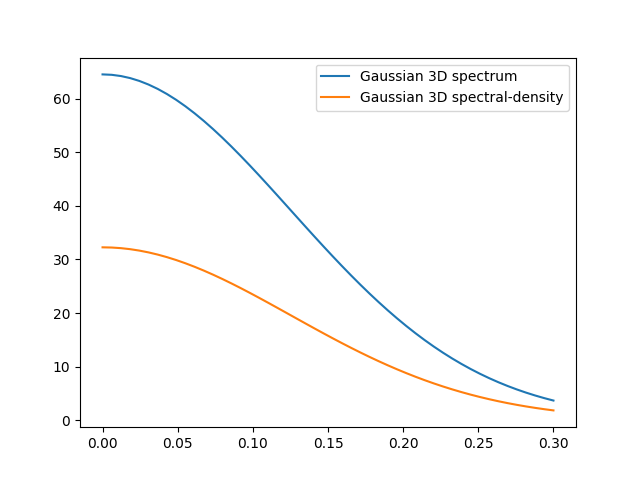# Spectral methods¶

The spectrum of a covariance model is given by:Since the covariance functionis radially symmetric, we can calculate this by the hankel-transformation:Where.

Depending on the spectrum, the spectral-density is defined by:You can access these methods by:

import gstools as gs

model = gs.Gaussian(dim=3, var=2.0, len_scale=10)
ax = model.plot("spectrum")
model.plot("spectral_density", ax=ax)Note

The spectral-density is given by the radius of the input phase. But it is not a probability density function for the radius of the phase. To obtain the pdf for the phase-radius, you can use the methods CovModel.spectral_rad_pdf or CovModel.ln_spectral_rad_pdf for the logarithm.

The user can also provide a cdf (cumulative distribution function) by defining a method called spectral_rad_cdf and/or a ppf (percent-point function) by spectral_rad_ppf.

The attributes CovModel.has_cdf and CovModel.has_ppf will check for that.

Total running time of the script: ( 0 minutes 0.115 seconds)

Gallery generated by Sphinx-Gallery